Qlik Sense App Development

Highlighted
New Contributor II

How to show recent a 3 months regression line in a 12 months bar chart

How to set up a regression line or trend line show the recent 3 months in a 12 months bar chart?

In particular, below graph show me all possible 12 months, but I only want to take show 3 months trend line? What is the possible solution?

linest_m(total aggr(if(sum([Cash_Payments]),sum([Cash_Payments])),Month),Month)*

+linest_b(total aggr(if(sum([Cash_Payments]),sum([Cash_Payments])),Month),Month)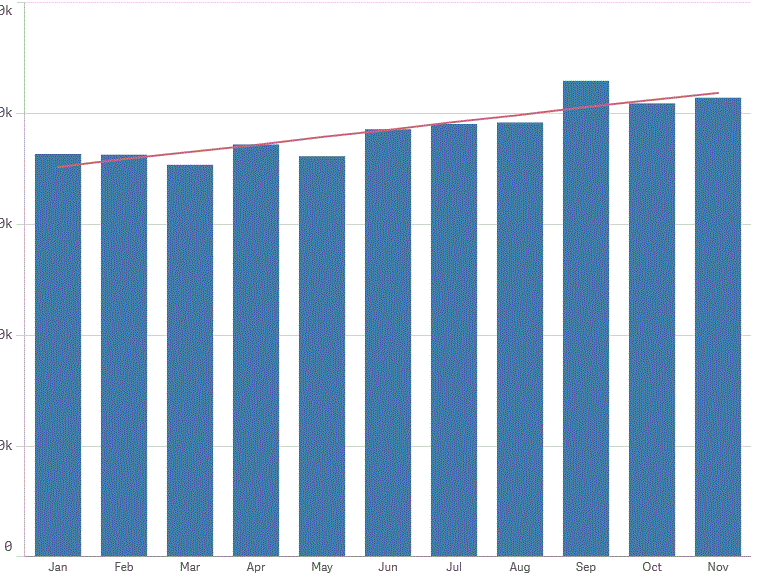Tags (3)
16 Replies
Valued Contributor III

Re: How to show recent a 3 months regression line in a 12 months bar chart

Hi,

Let's see if the below script helps you:

Basically, Date(Today(), 'MMM') pulls the today's date in months and Date(AddMonths(Today(),-3), 'MMM') looks back three months and pulls the month name:Hope this helps.

Thanks

New Contributor II

Re: How to show recent a 3 months regression line in a 12 months bar chart

Hi Sinan,

When I entered the formula, it return a red line only.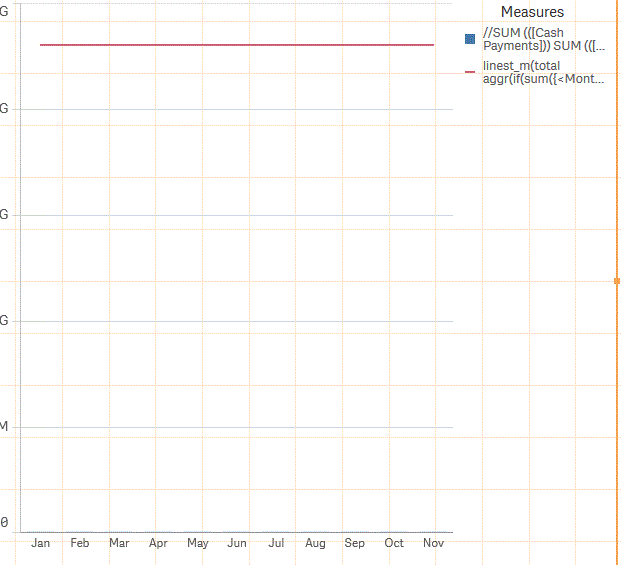Valued Contributor III

Re: How to show recent a 3 months regression line in a 12 months bar chart

Hi,

Do you happen to have sample data so I can try different things?

Thanks

New Contributor II

Re: How to show recent a 3 months regression line in a 12 months bar chart

sinanozdemir‌. Hi Sinan. Please see attached sample data. One correction, is it possible to create a regression line based on most recent, available 3 month data? Thanks so much for your help.

Valued Contributor III

Re: How to show recent a 3 months regression line in a 12 months bar chart

Hi,

So I got R2 and Ax numbers right for the last 3 months, let me know if this is something that you are looking for

BTW, you need to use numerical values of the months.In the bar chart, I created another expression just to show the last three months cash payments and turned on the trendlines to show linear.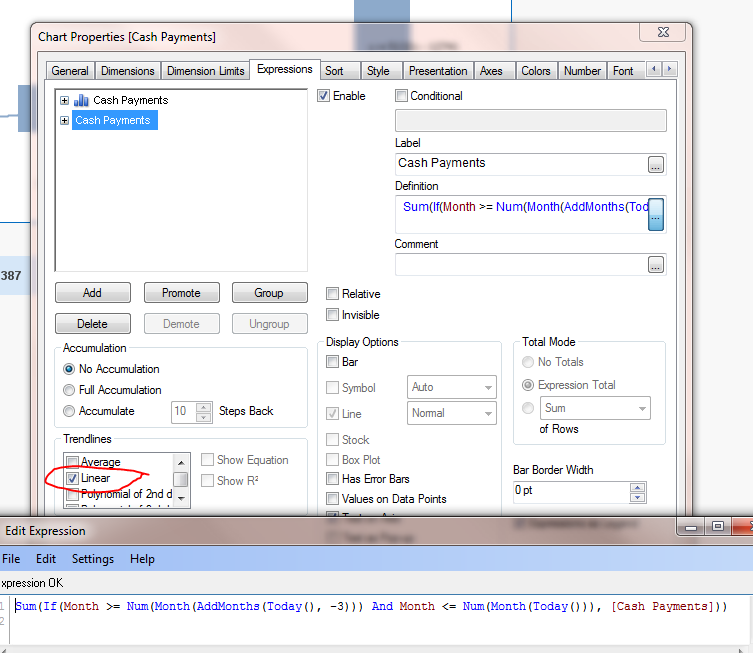I am also attaching the app and I hope this helps you.

Thanks

New Contributor II

Re: How to show recent a 3 months regression line in a 12 months bar chart

Yes. Thank you.

New Contributor II

Re: How to show recent a 3 months regression line in a 12 months bar chart

One last question. I entered the formula in the measurement, and what formula should I use 3 months trend line?

Valued Contributor III

Re: How to show recent a 3 months regression line in a 12 months bar chart

Hi,

Well, The way how I did was to create another expression which was Sum([Cash Receipts]) and unchecked the bar box. After that for that expression, I chose to show the linear trend line.

Look at my qvw to see how I did it.

Thanks

Valued Contributor III

Re: How to show recent a 3 months regression line in a 12 months bar chart

Ok. So I created a combo chart. Bars are showing the cash payments and the line is showing the linear trend for the last three months. I also put a straight table showing the formula and the trend lines in numeric values: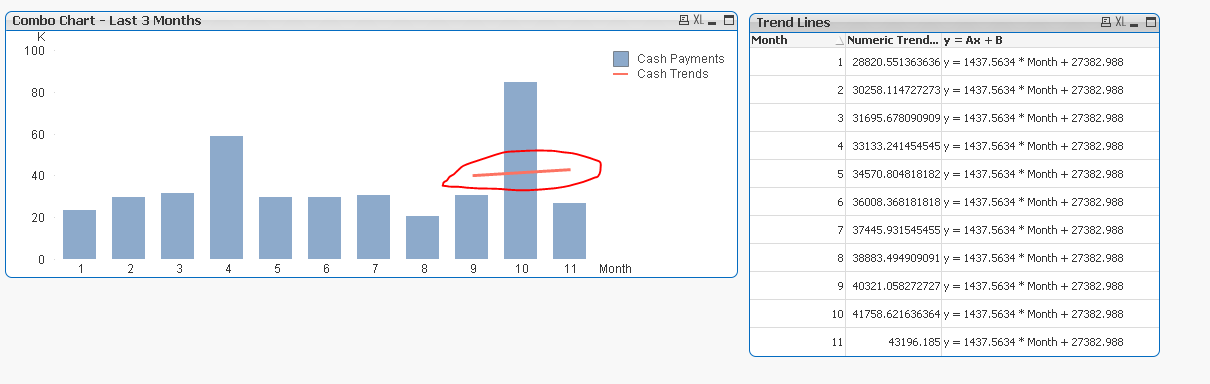The formula for the trend line is: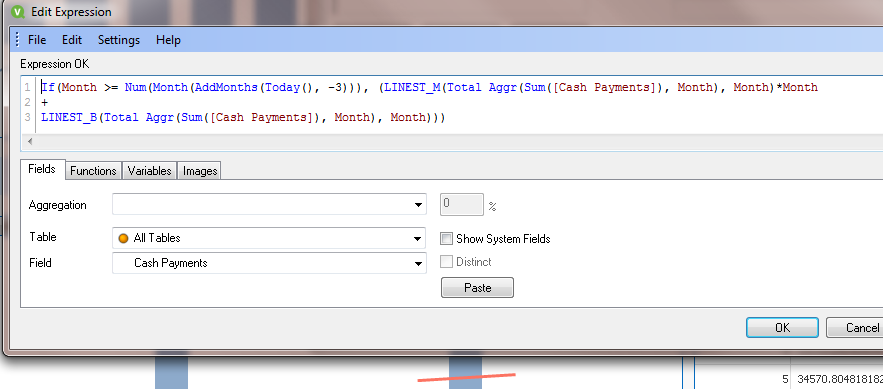I am attaching the app.